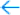# Automatic Experiment Tracking and Versioning

Part of your job as Data Scientist or Machine Learning engineer is to dilligently keep track of all the experiments you run. The problem is that doing that is not always easy with the tools and processes we tend to use.
Aurum will keep track of your experiments without requiring a change in your development workflow. After each execution of a new experiment, Aurum automatically collects all the parameters, code, metrics, and metadata generated in the process and saves this experiment inside git.
``````import aurum as au

au.use_datasets("dataset.csv")
au.parameters(test_size=0.15,
random_state=0,
n_estimators=1000)

# EXPERIMENT CODE

au.register_metrics(metric1=metric1,
metric2=metric2, ...)
au.end_experiment()``````
With Aurum you can also execute multiple instances of the same code with different parameters in parallel, and still record the performance of every experiment separatelly in git.
``````au.parameters(random_state=0,
n_estimators=1000)

model = RandomForestRegressor(n_estimators=au.n_estimators,
random_state=au.random_state)
model.fit(X_train, y_train)
y_pred = model.predict(X_test)
error = mean_absolute_error(y_test, y_pred)

au.register_metrics(mae=error)
au.end_experiment()``````
By executing a complete experiment with registered aurum parameters such as the ones found in the above example, you'll execute the experiment with the default values or with the ones provided during the call. The example bellow shows how the parameters would be passed to your script during the call:
``\$ python experiment.py n_estimators=500 random_state=1``
That not only makes for easy experimentation, it also makes for easier hyperparameter tunning while at the same time keeping track of your experiments. That is exactly why you should go to the projects Github pageright now, download and give it a try.Stripping